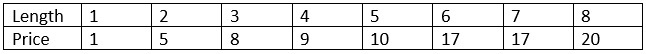# Rod Cutting

Dynamic ProgrammingData StructureAlgorithms

A rod is given of length n. Another table is also provided, which contains different size and price for each size. Determine the maximum price by cutting the rod and selling them in the market.

To get the best price by making a cut at different positions and comparing the prices after cutting the rod.

Let the f(n) will return the max possible price after cutting a row with length n. We can simply write the function f(n) like this.

f(n) := maximum value from price[i]+f(n – i – 1), where i is in range 0 to (n – 1).

## Input and Output

Input:

The price of different lengths, and the length of rod. Here the length is 8.Output:

Maximum profit after selling is 22.

Cut the rod in length 2 and 6. The profit is 5 + 17 = 22

## Algorithm

rodCutting(price, n)

Input: Price list, number of different prices on the list.

Output: Maximum profit by cutting rods.

Begin
define profit array of size n + 1
profit := 0
for i := 1 to n, do
maxProfit := - ∞
for j := 0 to i-1, do
maxProfit := maximum of maxProfit and (price[j] + profit[i-j-1])
done

profit[i] := maxProfit
done
return maxProfit
End

## Example

#include <iostream>
using namespace std;

int max(int a, int b) {
return (a > b)? a : b;
}

int rodCutting(int price[], int n) {    //from price and length of n, find max profit
int profit[n+1];
profit = 0;
int maxProfit;

for (int i = 1; i<=n; i++) {
maxProfit = INT_MIN;    //initially set as -ve infinity
for (int j = 0; j < i; j++)
maxProfit = max(maxProfit, price[j] + profit[i-j-1]);
profit[i] = maxProfit;
}
return maxProfit;
}

int main() {
int priceList[] = {1, 5, 8, 9, 10, 17, 17, 20};
int rodLength = 8;
cout << "Maximum Price: "<< rodCutting(priceList, rodLength);
}

## Output

Maximum Price: 22